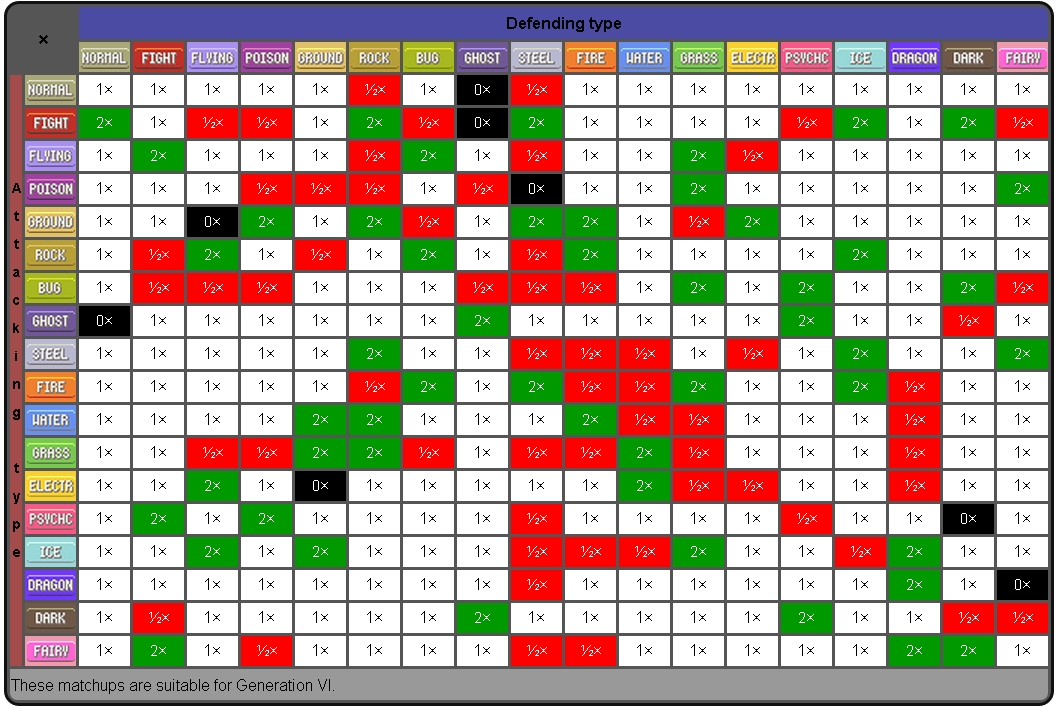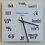# Pokémon Damage Formula

$$Damage = \dfrac{\left \lfloor{ \left[ \frac{2 \times Level_{1} + 10}{250} \times BasePwr \times \frac{ \left\{ \left( 2 \times BaseAtk + IV_{1} + \frac{EV_{1}}{4} \right) \times \frac{Level_{1}}{100} + 5 \right\} \times Nature_{1} \times AtkStatChange}{ \left\{ \left( 2 \times BaseDef + IV_{2} + \frac{EV_{2}}{4} \right) \times \frac{Level_{2}}{100} + 5 \right\} \times Nature_{2} \times DefStatChange} +2 \right] \times STAB \times Mod \times \frac{RanInt(85,100)}{100} }\right \rfloor}{\left( 2 \times BaseHP + IV^{\prime}_2 + \frac{EV^{\prime}_2}{4} \right) \times \frac{Level_{2}}{100} + 10 + Level_{2}} \times 100\%$$

$Where$

$P1\,=\,Attacking\,Pok\acute{e}mon;\,P2\,=\,Defending\,Pok\acute{e}mon$

$Level_{1}\,= \,Level\,of\,P1;\,Level_{2}\,=\,Level\,of\,P2,$

$BasePwr\,=\,Power\,of\,selected\,move,$

$BaseAtk,\,BaseDef\,=\,Base\,Attack\,of\,P1\,and\,Base\,Defense\,\,of\,P2\,respectively,$

$IV_{1},\,EV_{1}\,=\,Attack\,IVs\,and\,EVs\,of\,P1\,respectively,$

$IV_{2},\,EV_{2}\,=\,Defense\,IVs\,and\,EVs\,\,of\,P2\,respectively,$

$BaseHP,\,IV^{\prime}_{2},\,EV^{\prime}_{2}\,=\,Base HP,\,HP\,IVs\,and\,HP\,EVs\,of\,P2\,respectively,$

$RanInt(x, y) = \left\{ randomly\,choosen \, a \: | \: x \leq a \leq y, a \in Z \right\},$

$STAB\,= \left\{ \begin{array}{lrr} 1.5 & if & attack\,type\,matches\,with\,Pok\acute{e}mon\,type \\ 1 & if & attack\,type\,does\,not\,match\,with\,Pok\acute{e}mon\,type\end{array} \right. ,$

$AtkStatChange\,is\,the\,Attack\,stat\,modifier\,\,of\,P1\,and\,DefStatChange\,is\,the\,Defense\,stat\,modifier\,\,of\,P2 ,$

$Mod\,=\,type\,compatibility\,which\,can\,be\,deduced\,from\,the\,type\,chart .$

$Let\,a\,matrix$ $N_{5\times5}\,=\, \left[ \begin{array}{lllll} Hardy & Lonely & Adamant & Naughty & Brave \\ Bold & Docile & Impish & Lax & Relaxed \\ Modest & Mild & Bashful & Rash & Quiet \\ Calm & Gentle & Careful & Quirky & Sassy \\ Timid & Hasty & Jolly & Naive & Serious \end{array} \right]$

$Then,$

$Nature_{1} = \left\{ \begin{array}{lcr} 1.1 & if & \left\{ \begin{array}{lrr} attack\,is\,physical & and & i_{1}=1,\, j_{1}\neq1 \\ attack\,is\,special & and & i_{1}=2,\,j_{1}\neq2 \end{array} \right. \\ 1.0 & if & \left\{ \begin{array}{lrr} attack\,is\,physical & and & i_{1}\neq1,\, j_{1}\neq1 \\ \, & \, & i_{1} = j_{1} \\ attack\,is\,special & and & i_{1}\neq2,\,j_{1}\neq2 \end{array} \right. \\ 0.9 & if & \left\{ \begin{array}{lrr} attack\,is\,physical & and & i_{1}\neq1,\, j_{1}=1 \\ attack\,is\,special & and & i_{1}\neq2,\,j_{1}=2 \end{array} \right. \end{array} \right.$

$Nature_{2} = \left\{ \begin{array}{lcr} 1.1 & if & \left\{ \begin{array}{lrr} attack\,is\,physical & and & i_{2}=3,\, j_{2}\neq3 \\ attack\,is\,special & and & i_{2}=4,\,j_{2}\neq4 \end{array} \right. \\ 1.0 & if & \left\{ \begin{array}{lrr} attack\,is\,physical & and & i_{2}\neq3,\, j_{2}\neq3 \\ \, & \, & i_{2} = j_{2} \\ attack\,is\,special & and & i_{2}\neq4,\,j_{2}\neq4 \end{array} \right. \\ 0.9 & if & \left\{ \begin{array}{lrr} attack\,is\,physical & and & i_{2}\neq3,\, j_{2}=3 \\ attack\,is\,special & and & i_{2}\neq4,\,j_{2}=4 \end{array} \right. \end{array} \right.$

$Where \left( i_{1},j_{1}\right) and \left( i_{2},j_{2}\right)\,are\,the\,positions\,\,of\,the\,natures\,of\,P1\,and\,P2\,in\,N_{5\times5}\,respectively.$

$\left( e.g.\, the\,position\,of\,Impish\,nature\,in\,N_{5\times5}\,is\, (2,3) \right) \$/extract_itex] $For\,Pok\acute{e}mon\,Showdown\,(i.e.\,Level=100\,and\,all\,IVs\,=31\,the\,simplified\,formula\,is$ $Damage = \frac{\left \lfloor{ \left[ BasePwr \times \frac{ \left\{ 2 \times BaseAtk + 36 + \frac{EV_{1}}{4} \right\} \times Nature_{1} \times AtkStatChange}{ \left\{ 2 \times BaseDef + 36 + \frac{EV_{2}}{4} \right\} \times Nature_{2} \times DefStatChange} +2 \right] \times STAB \times Mod \times \frac{RanInt(85,100)}{100} }\right \rfloor}{\left( 2 \times BaseHP + 141 + \frac{EV^{\prime}_2}{4} \right) } \times 84\%$ $The\,type\,chart\,which\,will\,give\,the\,value\,of\,Mod:$Note by Muzaffar Ahmed 5 years, 7 months ago This discussion board is a place to discuss our Daily Challenges and the math and science related to those challenges. Explanations are more than just a solution — they should explain the steps and thinking strategies that you used to obtain the solution. Comments should further the discussion of math and science. When posting on Brilliant: • Use the emojis to react to an explanation, whether you're congratulating a job well done , or just really confused . • Ask specific questions about the challenge or the steps in somebody's explanation. Well-posed questions can add a lot to the discussion, but posting "I don't understand!" doesn't help anyone. • Try to contribute something new to the discussion, whether it is an extension, generalization or other idea related to the challenge. • Stay on topic — we're all here to learn more about math and science, not to hear about your favorite get-rich-quick scheme or current world events. MarkdownAppears as *italics* or _italics_ italics **bold** or __bold__ bold - bulleted- list • bulleted • list 1. numbered2. list 1. numbered 2. list Note: you must add a full line of space before and after lists for them to show up correctly paragraph 1paragraph 2 paragraph 1 paragraph 2 [example link](https://brilliant.org)example link > This is a quote This is a quote  # I indented these lines # 4 spaces, and now they show # up as a code block. print "hello world" # I indented these lines # 4 spaces, and now they show # up as a code block. print "hello world" MathAppears as Remember to wrap math in $$ ... $$ or \[ ... $ to ensure proper formatting.
2 \times 3 $2 \times 3$
2^{34} $2^{34}$
a_{i-1} $a_{i-1}$
\frac{2}{3} $\frac{2}{3}$
\sqrt{2} $\sqrt{2}$
\sum_{i=1}^3 $\sum_{i=1}^3$
\sin \theta $\sin \theta$
\boxed{123} $\boxed{123}$

Sort by:I salute you

- 5 years, 7 months ago

That is a: $\Huge{\color{#D61F06}{\text{HELL!!!}}}$ of a lot of LaTeX to write.

- 5 years, 7 months ago

Yeah lol I know.

Also yeah it took 4 hours straight to write that

- 5 years, 7 months ago

$\Huge{\color{#3D99F6}{\text{I salute you for your determination}}}$

- 5 years, 7 months ago

i play too!! did you try this. great battle simulator.

- 5 years, 7 months ago

Yeah I play on PS, everyday. :)

- 5 years, 7 months ago

same here.

- 5 years, 7 months ago

Up for a match right now?

- 5 years, 7 months ago

sure, i might not able to chat though... i got locked for spamming... knock me under the name dragonite drake. lets play AG.

- 5 years, 7 months ago

I was at 1590 before the ladder reset.. I left AG after that.. Do you play OU though?

- 5 years, 7 months ago

sometimes, i main in AG. my rating was 1801 before reset.

- 5 years, 7 months ago

i use to use 2 ferros at that time.

- 5 years, 7 months ago

u can see me at 90 on ladder with 1658... calls for rematch

- 5 years, 7 months ago